Reference:

• D. Schuurmans, Machine Learning course notes, University of Waterloo, 1999.
• T. Mitchell, Machine Learning, McGraw-Hill, 1997, pp. 20-50.

Machine learning is a process which causes systems to improve with experience.

Representation

1. Items of Experience
• iI
2. Space of Available Actions
• aA
3. Evaluation
• v (a, i)
4. Base Performance System
• b: I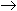A
5. Learning System
• L: (i1, a1, v1)...(in, an, vn)b
• Maps training experience sequence to base performance system.

Types of Learning Problems

Fundamental Distinctions

• Between problems rather than methods.
• Influences choice of method.
1. Batch versus Online Learning:
• Batch Learning
• Training phase (no responsibility for performance during learning), then testing.
• Synthesizing a base performance system.
• Pure exploration.
• Online Learning
• No separate phase.
• Learning while doing.
• Have to decide between choosing actions to perform well now versus gaining knowledge to perform well later.
2. Learning from Complete versus Partial Feedback:
• Complete Feedback
• Each item of experience evaluates every action (or base system).
• Tells what best action would have been.
• Partial Feedback
• Each item of experience evaluates only a subset of possible actions (or base systems).
• Only tells you how you did.
• Creates exploration/exploitation tradeoff in online setting.
• Should the reward be optimized with what is already known or should learning be attempted?
• In batch learning, exploration would be chosen since there is no responsibility for performance.
• Pointwise Feedback
• Evaluates single action.
• Optimization problem: (Controller, State)Action.
3. Passive versus Active Learning
• Passive: observation.
• Active: experimentation, exploitation.
4. Learning in Acausal versus Causal Situations
• Acausal: actions do not affect future experience.
• e.g., rain prediction
• Causal: actions do effect future experience.
5. Learning in Stationary versus Nonstationary Environments
• Stationary: evaluation doesn't change.
• Nonstationary: evaluation changes with time.

Concept Learning

The problem of inducing general functions from specific training examples is central to learning.

Concept learning acquires the definition of a general category given a sample of positive and negative training examples of the category, the method of which is the problem of searching through a hypothesis space for a hypothesis that best fits a given set of training examples.

A hypothesis space, in turn, is a predefined space of potential hypotheses, often implicitly defined by the hypothesis representation.

Learning a Function from Examples

• An example of concept learning where the concepts are mathematical functions.

Given:

• Domain X: descriptions
• Domain Y: predictions
• H: hypothesis space; the set of all possible hypotheses
• h: target hypothesisIdea: to extrapolate observed y's over all X.

Hope: to predict well on future y's given x's.

Require: there must be regularities to be found!

(Note type: batch, complete, passive (we are not choosing which x), acausal, stationary).

Many Research Communities

• Representation choice differs.
• Prefer maximum level of abstraction.

• h: RnR
• Squared prediction error.
• h is a linear function.

• h: R{0, 1}
• Right/wrong prediction error.
• h is a linear discriminant boundary.

"Symbolic" Machine Learning

• h: {attribute-value vectors}{0, 1}
• h is a simple boolean function (eg. a decision tree).

Neural Networks

• h: RnR
• h is a feedforward neural net.

Inductive Logic Programming

• h: {term structure}{0, 1}
• h is a simple logic program.

Learning Theory

• Postulate mathematical model of learning situations.
• Rigorously establish achievable levels of learning performance.
• Characterize/quantify value of prior knowledge and constraints.
• Identify limits/possibilities of learning algorithms.

Standard Learning Algorithm

Given a batch set of training data S = {(x1, y1)...(xt, yt)}, consider a fixed hypothesis class H and compute a function hH that minimizes empirical error.Example: Least-Squares Linear Regression

Here, the standard learning algorithm corresponds to least squares linear regression.

X = Rn, Y = R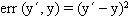H = {linear functions RnR}

Choosing a Hypothesis Space

In practice, for a given problem XY, which hypothesis space H do we choose?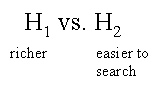Question: since we know the true function f: XY, should we make H as "expressive" as possible and let the training data do the rest?

• e.g., high degree polynomial
• e.g., complex neural network.

Reason: overfitting!

• Underfit
• Unable to express a good prediction.
• Overfit
• Hard to identify good from bad candidates.

Basic Overfitting Phenomenon

Example: polynomial regression

Suppose we use a hypothesis space, with many classes of functionsGiven n = 10 training points (x1, y1)...(x10, y10).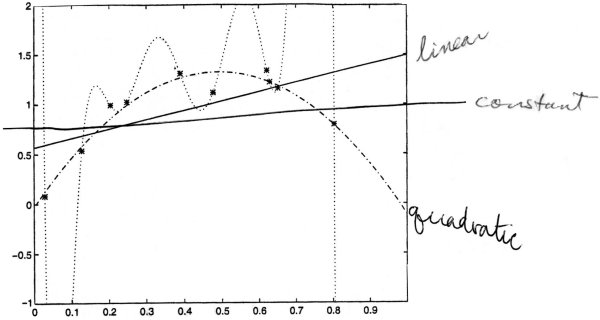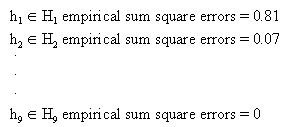Since n = 10, with 10 pieces of data, then in

Hn - 1 = H9

there is a function that gives an exact fit.

Which hypothesis would predict well on future examples?

• Although hypothesis h9 fits these 10 data exactly, it is unlikely to fit the next piece of data well.

When does empirical error minimization overfit?

Depends on the relationship between

• hypothesis class, H
• training sample size, t
• data generating process